# 2一般基坑加载状态时内撑式基坑支护变形研究

## 2.1内撑式基坑土压力计算理论

《建筑基坑支护技术规程 JGJ120-2012》给出了水土合算和水土分算的土压力计算公式：

$${p_{ak}} = {\sigma _{ak}}{K_{a,i}} - 2{c_i}\sqrt {{K_{a,i}}}$$ $${K_{a,i}} = {\tan ^2}(45 - \frac{{{\varphi _i}}}{2})$$ $${p_{pk}} = {\sigma _{pk}}{K_{p,i}} - 2{c_i}\sqrt {{K_{p,i}}}$$ $${K_{p,i}} = {\tan ^2}(45 + \frac{{{\varphi _i}}}{2})$$

$${p_{ak}} = ({\sigma _{ak}} - {u_a}){K_{a,i}} - 2{c_i}\sqrt {{K_{a,i}}} + {u_a}$$ $${p_{pk}} = ({\sigma _{pk}} - {u_p}){K_{p,i}} + 2{c_i}\sqrt {{K_{p,i}}} + {u_p}$$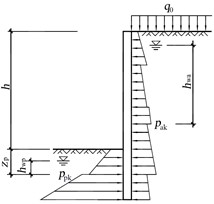“规范”中规定的土压力计算方法为计算静土压力的方法，当支护结构受到土压力发生变形之后，净土压力即转变为主动土压力和被动土压力，土压力的大小和计算方法发生变化。而当前土力学理论中计算主动土压力和被动土压力的方法主要为朗肯土压力理论和库伦土压力理论，已有的理论计算方法均是假定土体位移已经达到极限状态。在实际工程中，主动土压力的位移极限状态较为容易达到，被动土压力的位移极限状态由于需要较大的土体位移，往往超过工程允许范围。因此有不少研究者针对土体位移未达到极限状态时的土压力理论进行了相关研究，当前常用的土体位移修正示意图如下图所示：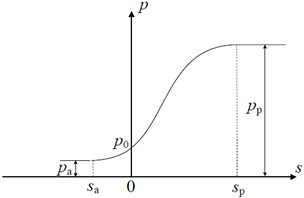RB模式下的主动土压力计算公式：

$${p_h} = \left\{ {\begin{array}{*{20}{c}} {{K_1}\gamma z(0 \le z \le {h_1})}\\ {{K_2}\gamma (z - {h_1}) + {K_1}\gamma {h_1}({h_1} < z \le H)} \end{array}} \right.$$

$${K_1} = \frac{{\sin (\alpha - \varphi )\cos \delta \cot \alpha }}{{\cos (\alpha - \varphi - \varphi )}}$$ $${K_2} = \frac{{\cos (\alpha - \varphi + \delta )}}{{\cos (\alpha - \varphi - \delta )}}{K_1}$$ $${h_1} = B\tan \alpha$$

$${\alpha _{cr}} = \arctan \left[ {\sqrt {\frac{{\tan \varphi }}{{\tan (\varphi + \delta )}} + {{\tan }^2}(\varphi ) + \tan (\varphi )} } \right]$$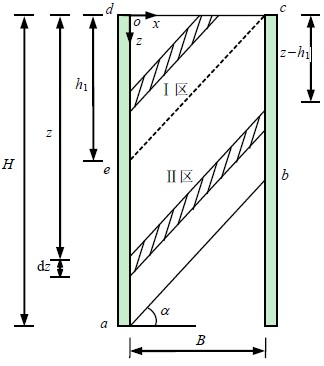RB模式下的被动土压力计算公式为
$${p_{RB}} = ({K_p} - {K_0})\gamma \left( {\frac{3}{{2 - 3m}}\frac{{{z^2}}}{H} - \frac{{3m}}{{2 - 3m}}z} \right) + {K_0}\gamma z$$

$${K_p} = \frac{{{{\cos }^2}\varphi }}{{{{(\sqrt {\cos \delta } - \sqrt {\sin (\delta + \varphi )\sin \varphi } )}^2}}}$$

T模式下的未达到极限状态和极限状态的被动土压力的计算公式：

$${p_T} = \left\{ {\begin{array}{*{20}{c}} {\left[ {\left( {{K_p} - K_0} \right)\frac{s}{{{s_p}}} + {K_0}} \right]\gamma z}&{s \le {s_p}}\\ {{K_p}\gamma z}&{s > {s_p}} \end{array}} \right.$$

RBT模式下的被动土压力计算公式如下式所示：

$${p_{RBT}} = \left\{ {\begin{array}{*{20}{c}} {{K_p}\gamma z}&{0 \le z \le (1 - \frac{{{s_p}}}{{{s_{\max }}}})H}\\ {\left( {{K_0} - ({K_0} - K{'_p})\frac{{{s_{\max }}}}{{{s_p}}}\frac{{H - z}}{H}} \right)\gamma z}&{(1 - \frac{{{s_p}}}{{{s_{\max }}}})H \le z \le H} \end{array}} \right.$$

$$K{'_p} = - \frac{2}{{1 + 3n}}{K_0} + \frac{{3 + 3n}}{{1 + 3n}}{K_p}$$

RTT模式下的被动土压力计算公式如下式所示

$${p_{RTT}} = \left\{ {\begin{array}{*{20}{c}} {(K{'_p} - {K_0})\frac{{z + nH}}{{(n + 1)H}}\frac{{{s_{\max }}}}{{{s_p}}}\gamma z + {K_0}\gamma z}&{{s_{\max }} \le {s_p}}\\ {\begin{array}{*{20}{c}} {\left( {{K_0} - ({K_0} - K{'_p})\frac{{{s_{\max }}}}{{{s_p}}}\frac{{z + nH}}{{(n + 1)H}}} \right)\gamma z}&{0 \le z \le (n + 1)\frac{{{s_p}}}{{{s_{\max }}}}H - nH}\\ {{K_p}\gamma z}&{(n + 1)\frac{{{s_p}}}{{{s_{\max }}}}H - nH \le z \le H} \end{array}}&{{s_{\max }} > {s_p}} \end{array}} \right.$$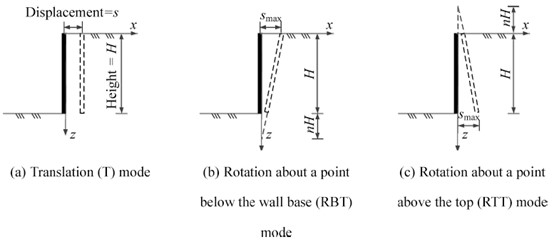## 2.2内撑式基坑支护结构变形理论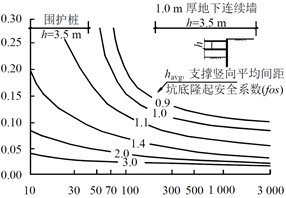1. 在安装第一道钢支撑前，桩身变形桩顶较小，桩底变形较大，排桩呈直线向基坑内偏移。施工第二道钢支撑后，桩身变形整体呈“凸”型，且桩顶位移较小，桩底位移向基坑外偏移较大，中部向坑内变形。

2. 钢支撑的施加能够控制排桩向基坑内的变形。钢支撑轴力模拟值与监测值接近，而设计值偏大；排桩弯矩、剪力监测值与设计结果较为吻合。

3. 增加钢支撑刚度、预加轴力和排桩自身刚度，可以有效的抑制排桩水平位移，同时也会使得排桩弯矩增加，内支撑轴力逐渐减小。

4. 随着泥岩粘聚力、内摩擦角、弹性模量减小或泊松比的增加，排桩中下部位移都会一定程度的增加。其中，泥岩泊松比对排桩的水平位移的影响最大，其次为弹性模量、内聚力、内摩擦角。

# 3非对称开挖内撑式基坑支护结构变形的共性与个性

## 3.2非对称内撑式支护结构变形的个性

### 3.2.1非对称支护结构的变形特性

1. 挖深较深一侧的支护结构平动变形更大，同时随着平动的增加横向支撑的等效轴向刚度逐渐减小；而在挖深较浅的一侧，随着平动变形的增加，支撑的不动点系数逐渐减小。且随着挖深的增加，挖深较深侧的支护结构变形持续增大，挖深较浅侧的支护结构变形增大较缓。

2. 在非对称开挖基坑支护的设计中可以通过调整截面的尺寸和嵌固深度来减小非对称变形对刚度和稳定性变化的影响。

3. 两侧挖深差对开挖较深侧坑外地表沉降影响很大，当两侧挖深差增大时开挖较深侧坑外地表沉降会急剧增大；而开挖较浅侧坑外地表沉降会接近线性增大，且增量较小。

4. 开挖分界面对坑底中间部分隆起的影响较大，而对两边靠近支护结构的隆起的影响很小，且隆起最大值的位置不随开挖分界面的改变而改变。随着两侧荷载差的不断增大，两侧坑外地表沉降的差值也不断增大，与相应对称开挖的差值也越大；随着非对称荷载位置距基坑的距离不断增大，两侧坑外地表沉降的变化较小。

### 3.2.2非对称支护结构的受力特性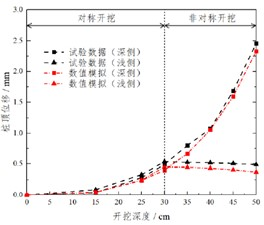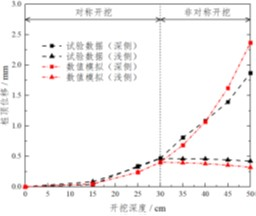# 4小结

1. 基坑开挖的后，作用在支护结构上的土压力往往是非极限状态的，因此在计算时应当考虑做非极限状态进行设计。

2. 内撑式基坑支护变形理论的核心是支护结构的变形刚度。其中当前大部分的基坑支护结构的变形刚度理论均基于Clough综合刚度模型推导得到，且根据不同的工况存在不同的计算变体。

3. 一般基坑支护结构的内力和变形与非对称开挖状态下的内力变形主要有两大共同点：（1）土压力的计算方法相同；（2）支护结构的刚度模型相似。

4. 非对称开挖状态下的基坑支护变形特性的主要特性有：不同挖深侧的支护结构变形特性不同；受力特性主要包括不同变形差异的横向支撑推回作用。

# 参考文献

1. 中国建筑科学研究院, 建设综合勘察设计研究院, 北京市勘察设计研究院, et al. 建筑地基基础设计规范 [M]. 中华人民共和国国家标准. 2011: 347p:A4.
2. 罗志元. 内撑式非对称开挖条件下的基坑变形研究 [D]; 浙江大学, 2012.
3. 殷铭. 非对称开挖基坑内撑式围护结构受力及变形分析 [D]; 浙江大学, 2016.
4. 中国建筑科学研究院, 中冶建筑研究总院有限公司, 华东建筑设计研究院有限公司, et al. 建筑基坑支护技术规程 [M]. 行业标准-建筑工业. 2012: 220P.;B5.
5. 李平, 杨挺, 王义, et al. 基坑工程隆起变形研究综述 [J]. 河海大学学报: 自然科学版, 2010, 38(2): 196-201.
6. 杨光华. 土钉支护技术的应用与研究进展 [J]. 岩土工程学报, 2010, 32(1): 9-16.
7. XIAO J, DAI F, WEI Y, et al. Analysis of mechanical behavior in a pipe roof during excavation of a shallow bias tunnel in loose deposits [J]. Environmental Earth Sciences, 2016, 75(4): 293.
8. XU C, XU Y, SUN H, et al. Characteristics of braced excavation under asymmetrical loads [J]. Mathematical problems in engineering, 2013, 2013(
9. 李广信. 基坑支护结构上水土压力的分算与合算 [J]. 岩土工程学报, 2000, 22(3): 348-52.
10. 陈愈炯, 温彦锋. 基坑支护结构上的水土压力 [J]. 岩土工程学报, 1999, 21(2): 4-8.
11. CONTE E, TRONCONE A, VENA M. A method for the design of embedded cantilever retaining walls under static and seismic loading [J]. Geotechnique, 2017, 67(12): 1081-9.
12. LIU, H. Required reinforcement stiffness for vertical geosynthetic-reinforced-soil walls at strength limit state [J]. Geotechnique, 2016,
13. MING-XI O U, LIU X R, SHI J X. Earth pressure applied in retaining wall of deep foundation pit considering influence of unloading and deformation [J]. Journal of Central South University(Science and Technology), 2012, 43(2): 669-74.
14. 张恒志, 徐长节, 梁禄钜, et al. RB 模式下刚性挡墙有限土体主动土压力的 离散元模拟与理论研究 [J]. 岩土力学, 42(10): 2895-907.
15. FAN D-L, HUANG L. The Effect and Application of Spatial Effect on the Asymmetrical Deep Excavation [J]. Electronic Journal of Geotechnical Engineering, 2016, 21(1063-78.
16. PENG S Q, XI-BING L I, FAN L, et al. A general method to calculate passive earth pressure on rigid retaining wall for all displacement modes [J]. Transactions of Nonferrous Metals Society of China, 2012, 22(6): 1526-32.
17. FAN X-Z, XU C-J, LIANG L-J, et al. Analytical solution for displacement-dependent passive earth pressure on rigid walls with various wall movements in cohesionless soil [J]. Computers and Geotechnics, 2021, 140(104470.
18. TURNER M J, CLOUGH R W, MARTIN H C, et al. Stiffness and deflection analysis of complex structures [J]. journal of the Aeronautical Sciences, 1956, 23(9): 805-23.
19. CLOUGH G W, SMITH E M, SWEENEY B P. Movement control of excavation support systems by iterative design; proceedings of the Foundation engineering: Current principles and practices, F, 1989 [C]. ASCE.
20. 周松. 深基坑内撑式排桩支护结构变形及内力研究 [J]. 成都理工大学, 2017, 1-z.
21. GAO X, TIAN W-P, ZHANG Z. Analysis of deformation characteristics of foundation-pit excavation and circular wall [J]. Sustainability, 2020, 12(8): 3164.
22. GIRGIDOV A. The time of groundwater free surface lowering before foundation pit construction [J]. Magazine of Civil Engineering, 2012,
23. JC L. Use of 3D finite element method for back study of a failed basement excavation in soft clay [J]. Japanese Geotechnical Society Special Publication, 2016, 2(73): 2504-7.
24. KANG C, XU R, YING H W, et al. Simplified method for calculating ground lateral displacement induced by foundation pit excavation [J]. Engineering Computations, 2020, ahead-of-print(ahead-of-print):
25. MIAN L, YANG X, BO Z. RESEARCH ON LATERAL STIFFNESS OF STRUTTED RETAINING STRUCTURE FOR DEEP EXCAVATION [J]. 工程地质学报, 2020, 28(5): 1116-22.
26. CHUNLIN H, LI C, RENAN L, et al. Monitoring technique and danger situation forecast during the excavation of deep foundation pit [J] [J]. Rock and Soil Mechanics, 1996, 2(
27. CHEN X, XU W, DUAN C. Numerical study on asymmetric excavation-induced supporting structure effect of∞-shape super deep foundation pit; proceedings of the 2011 International Conference on Electric Technology and Civil Engineering (ICETCE), F, 2011 [C]. IEEE.
28. 王宁. 深基坑开挖变形神经网络监测模型研究 [D]; 上海交通大学, 2009.
29. 徐长节, 殷铭, 胡文韬. 非对称开挖基坑支撑式围护结构解析解 [J]. 岩土力学, 2017, 38(08): 2306-12.
30. 尹骥, 管飞, 李象范. 直径210m超大圆环支撑基坑设计分析 [J]. 岩土工程学报, 2006, S1): 1596-9.
31. 张戈, 毛海和. 软土地区深基坑围护结构综合刚度研究 [J]. 岩土力学, 2016, 37(5): 8.
32. 王飞阳. 内撑式非对称基坑支护体系性状分析 [D]; 华南理工大学, 2016.
33. FAN X-Z, PHOON K-K, XU C-J, et al. Closed-form solution for excavation-induced ground settlement profile in clay [J]. Computers and Geotechnics, 2021, 137(104266.
34. 徐长节, 成守泽, 蔡袁强, et al. 非对称开挖条件下基坑变形性状分析 [J]. 岩土力学, 2014, 35(07): 1929-34.

https://www.eatrice.cn/post/AsymmetricalExcavation/

2021年12月2日